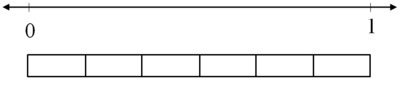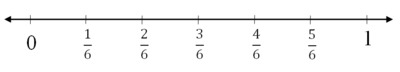# Folding Strips of Paper

Alignments to Content Standards: 5.NF.B.4

1. Label the points on the number line that correspond to $\frac16$, $\frac26$, $\frac36$, $\frac46$, and $\frac56$.2. Carefully cut out a strip of paper that has a length of $\frac56$.
1. Bring the ends of the strip together to fold the strip of paper in half. How long is half of the strip? Use your strip to mark this point on the number line.
2. What two numbers can you multiply to find the length of half the strip? Write an equation to show this.
3. Unfold your paper strip so that you start with $\frac56$ again. Now fold the strip of paper in half and then in half again.
1. How long is half of half of the strip? Use your strip to mark this point on the number line.
2. What numbers can you multiply to find the length of half the strip? Write an equation to show this.

## IM Commentary

The purpose of this task is to provide students with a concrete experience they can relate to fraction multiplication. Perhaps more importantly, the task also purposefully relates length and locations of points on a number line, a common trouble spot for students. This task is meant for instruction and would be a useful as part of an introductory unit on fraction multiplication.

The Standards for Mathematical Practice focus on the nature of the learning experiences by attending to the thinking processes and habits of mind that students need to develop in order to attain a deep and flexible understanding of mathematics. Certain tasks lend themselves to the demonstration of specific practices by students. The practices that are observable during exploration of a task depend on how instruction unfolds in the classroom. While it is possible that tasks may be connected to several practices, only one practice connection will be discussed in depth. Possible secondary practice connections may be discussed but not in the same degree of detail.Â

This particular task engages students in Mathematical Practice Standard 5, Use appropriate tools strategically. The students are directed to create a number line marking 1/6, 2/6, 3/6, 4/6, and 5/6.Â  Then they carefully cut out a strip of paper of 5/6 length and fold it in half and then fold it in half again. The paper folding along with the connection to the number line helps students visualize that when you divide a fraction in half, it is the same as multiplying Â½ by 5/6. Â The same thinking corresponds to folding the strip once again in half. Folding the paper in half twice is really the same as folding it into fourths or multiplying 1/4 by 5/6.

As students become proficient in MP.5, they will be able to consider a toolâ€™s usefulness and consider its strengths and limitations, as well as know how to use it appropriately. The solution pathway that a student selects needs to make sense to him/her to be able to explain and justify (MP.3). Students will come to realize that certain methods/tools are more efficient and they will abandon less useful tools in favor of more appropriate strategies/tools. Some tools might make more sense for a student to use depending on their level of understanding vs. their need for efficiency.Â  While some tools might make more sense for beginning to understand a concept, others might make more sense once students are searching to make calculations in the most efficient way.

## Solution

1.1. Half of the strip has a length of $\frac{5}{12}$; you can see this in the figure below. There are 5 sixths, so when you fold it in half, there are $2\frac12$ sixths in half the strip. Since half of a sixth is a twelfth, we can see how long the strip is by dividing every sixth in half and then seeing how many 12ths there are in half the strip.2. Half of $\frac56$ is the same as $\frac12 \times \frac56$. So folding the strip of paper in half will result in a strip of paper that is $$\frac{1}{2} \times \frac{5}{6} =\frac {5}{12}$$ in length.

1. Half of half of the strip has a length of $\frac{5}{24}$; you can see this in the figure below. Half of the strip has a length of $\frac{5}{12}$, so when you fold it in half, there are $2\frac12$ twelfths in half of half of the strip. Since half of a twelfth is a twenty-fourth, we can see how long the strip is by dividing every twelfth in half and then seeing how many 24ths there are in half of half of the strip.2. Half of half of $\frac56$ is the same as $\frac12 \times \frac12 \times \frac56 = \frac14 \times \frac56$. So folding the strip of paper in half and then in half again will result in a strip of paper that is

$$\frac{1}{4} \times \frac{5}{6} =\frac {5}{24}$$ in length.